#1

## SQL指令該如何寫?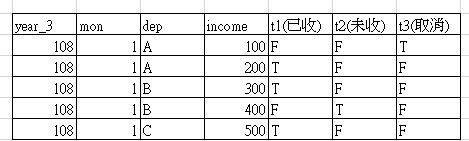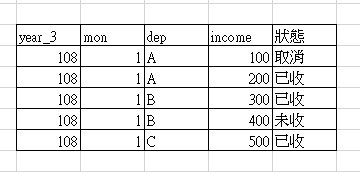### 4 個回答

6
``````SELECT year_3,mon,dep,income,
(CASE
WHEN t1='T' THEN N'已收'
WHEN t2='T' THEN N'未收'
WHEN t3='T' THEN N'取消'
END) AS '狀態'
FROM Temp
``````

Demo

hheyjen iT邦新手 5 級 ‧ 2019-07-11 13:52:30 檢舉

1

SQL有個關鍵字叫case

case when 應該對你不難寫吧

hheyjen iT邦新手 5 級 ‧ 2019-07-11 11:16:31 檢舉

case when不能轉成中文字串

hheyjen iT邦新手 5 級 ‧ 2019-07-11 11:34:26 檢舉

SELECT year_3,mon,dep,income,

(CASE
WHEN t1='TRUE' THEN '已收'
WHEN t2='TRUE' THEN '未收'
WHEN t3='TRUE' THEN '取消'
END) AS 狀態
FROM A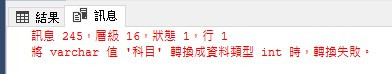player iT邦大師 1 級 ‧ 2019-07-11 11:35:22 檢舉

SQL 我只是在SQL Server 6.5時期才開始學的

``````SELECT year_3,mon,dep,income,

(CASE
WHEN t1=1 THEN '已收'
WHEN t2=1 THEN '未收'
WHEN t3=1 THEN '取消'
END) AS 狀態
FROM A
``````

dragonH iT邦大師 1 級 ‧ 2019-07-11 14:17:03 檢舉

it邦 sql查詢器

WQ iT邦新手 4 級 ‧ 2019-07-12 09:44:27 檢舉

WHEN t1='TRUE' THEN '已收' <==true 不要用''這個吧
WHEN t2='TRUE' THEN '未收'
WHEN t3='TRUE' THEN '取消'

2
``````SELECT
year3,mon,dep,income,
CASE WHEN t3=TRUE THEN '取消' ELSE
CASE WHEN t1=TRUE THEN '已收' ELSE '未收' END
END AS 狀態
FROM yourtable
WHERE    .........................
ORDER BY .........................
``````

※如果你的SQL版本不支援TRUE/FALSE，那改成t3=1(真)或0就可以

hheyjen iT邦新手 5 級 ‧ 2019-07-11 11:50:55 檢舉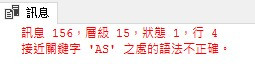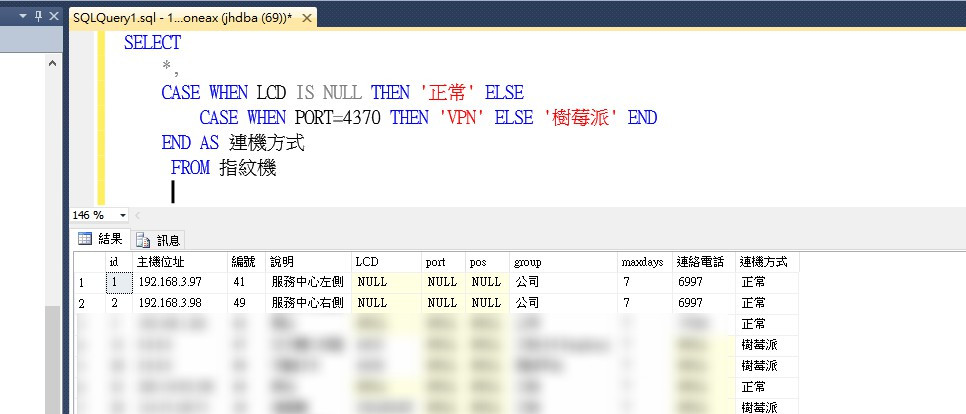0